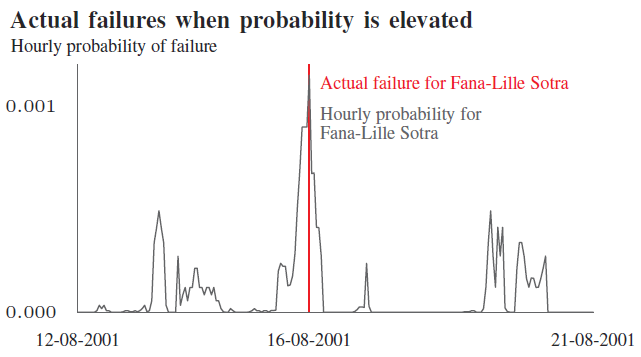Setting up a forecast service for weather dependent failures on power lines in one week and ten minutes

Combining Tableau, Python, Splunk and open data from met.no to deliver a realtime forecast of the probability of failure due to wind and lightning on overhead lines.

Our last post was about estimating the probability of failure for overhead lines based on past weather and failure statistics. The results are currently used as part of our long term planning process, by using a Monte Carlo tool to simulate failures in the Norwegian transmission system.

In this post you can read about how we re-used this model for a different use case: using the current weather forecast to predict probability of failure per overhead line. This information is very relevant for our system operators when preparing for severe weather events. We use data from the Norwegian Meteorological Institute, Tableau for visualization, Python for our backend and Splunk for monitoring. This post explains how.

Overview of the forecast service

The core delivery of the mathematical model described in our previous blog post are fragility curves per overhead line. To recall from the previous post, those fragility curves are constructed based on historical failures on overhead line combined with historical weather data for that line. The resulting fragility curves give us the relation between the weather event and the expected probability of failure. In our current model we have two fragility curves per line: one that describes the expected failure due to wind vs wind exposure, and one that models the expected failure due to lightning vs lightning exposure.

Now, when we have this information per overhead line, we can combine this with weather forecast data to forecast expected failure rates for all overhead lines. Our service downloads the latest weather forecast when it becomes available, updates the expected failure probabilities, and exports the results to file. We designed a Tableau dashboard to visualize the results in an interactive and intuitive manner.

The resulting setup is displayed below:In the remainder, we cover how we created and deployed this service.

Data input: fragility curves

See our previous blog post for an explanation of the creation of the fragility curves.

Data input: open weather data

The Norwegian Meteorological Institute (met.no) maintains a THREDDS server where anyone can download forecast data for Norway. THREDDS is an open source project which aims to simplify the discovery and use of scientific data. Datasets can be served through OPeNDAP, OGC’s WMS and WCS, HTTP, and other remote data access protocols. In this case we will use OPeNDAP so we don’t have to download the huge forecast files , only the variables we are interested in.

The first thing to notice is that we select the latest deterministic forecast meps_det_extracted_2_5km_latest.nc and load this using xarray. An alternative here would have been to download the complete ensamble forecast to get a sense of the uncertainty in the predictions as well. Next, we select wind_speed as the variable to load. Then we use the scipy.spatial.cKDTree package to find the nearest grid point to the ~30 000 power line towers and load the corresponding wind speed.

Forecast service: service creation and monitoring

We have developed both the probability model and the forecast service in Python. The forecasts are updated four times a day. To download the latest forecast as soon as it becomes available we continuously run a Windows process using Pythons win32service package. Only a few lines of code were necessary to do so:

The actual logic of obtaining the fragility curves, downloading new forecasts, and combining this into the desired time series is included in the download_service64Bit module.

To ensure the service behaves as desired, we monitor the process in two ways. Firstly, we use Splunk to monitor the server’s windows event log to pick up any unexpected crashes of the service. The team will receive an email within 15 minutes of an unexpected event. Secondly, we will develop more advanced analytics of our log using Splunk. This is made very easy when using Python’s excellent logging module (a great introduction on why and how you should use this module can be found here).

Publishing results to Tableau

We use Tableau as a quick and easy way to create interactive and visually attractive dashboards. An example screenshot can be seen below:In the top left corner, the information about the system state is shown. The percentage is the total probability of at least one line failing in the coming forecast period of three days. The dashboard shows the situation just before a major storm in January this year, leading to a very high failure probability. There actually occurred seven failures due to severe wind two days after the forecast. The bar plot shows which part of the estimated failure probability is due to wind versus lightning.

The main graph shows the development of failure over time for the whole power system. When no specific line is selected, this graph shows the development of the failure probability in the whole system over time:When one line is selected, it shows the details for this line: wind speed or lightning intensity, and estimated failure probability over time.The heat map at the bottom of the screen shows the probability of failure per overhead line per hour.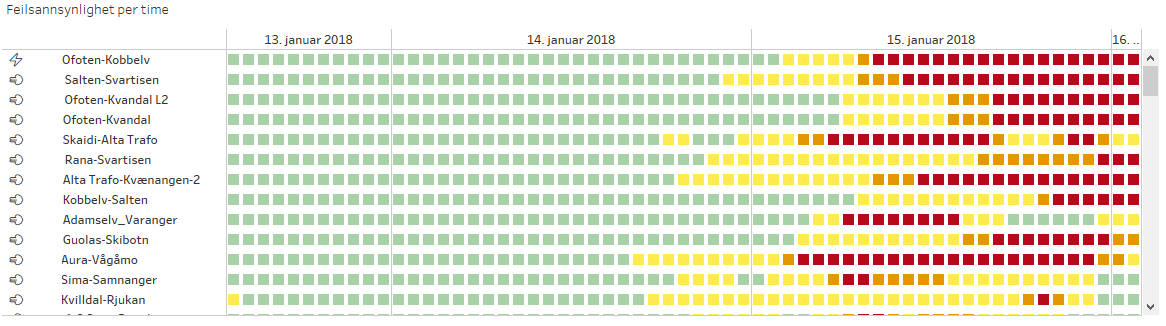The list of lines is ordered, listing the lines that are most likely to fail on top. This provides the system operator with a good indication of which lines to focus on. The heat map shows how the failure probability develops over time, showing that not all lines are exposed maximally at the same time. The wind/lightning emoji before the name of the line indicates whether that line is exposed to high wind or lightning.

Going from idea to deployment in one week and ten minutes

This weeks goal was to go from idea to deployment in one week by setting aside all other tasks. However, as Friday evening came closer, more and more small bugs kept popping up both in the data visualization as well as in the back-end.

In the end, we got the service at least 80% complete. To get the last things in place we might need just 10 minutes more…

Estimating the probability of failure for overhead lines

In Norway, about 90 percent of all temporary failures on overhead lines are due to weather. In this post, we present a method to model the probability of failures on overhead lines due to lightning.

Welcome to the blog for Data Science in Statnett, the Norwegian electricity transmission system operator. We use data science to extract knowledge from the vast amounts of data gathered about the power system and suggest new data-driven approaches to improve power system operation, planning and maintenance. In this blog, we write about our work. Today’s topic is a model for estimating the probability of failure of overhead lines.

Knowing the probability of failure is central to reliability management

For an electricity transmission system operator like Statnett, balancing power system reliability against investment and operational costs is at the very heart of our operation. However, a more data-driven approach can improve on the traditional methods for power system reliability management. In the words of the recently completed research project Garpur:

Historically in Europe, network reliability management has been relying on the so-called “N-1” criterion: in case of fault of one relevant element (e.g. one transmission system element, one significant generation element or one significant distribution network element), the elements remaining in operation must be capable of accommodating the new operational situation without violating the network’s operational security limits.

Today, the increasing uncertainty of generation due to intermittent energy sources, combined with the opportunities provided e.g. by demand-side management and energy storage, call for imagining new reliability criteria with a better balance between reliability and costs.

In such a framework, knowledge about failure probabilities becomes central to power system reliability management, and thus the whole planning and operation of the power system. When predicting the probability of failure, weather conditions play an important part; In Norway, about 90 percent of all temporary failures on overhead lines are due to weather, the three main weather parameters influencing the failure rate being wind, lightning and icing. In this post, we present a method to model the probability of failures on overhead lines due to lightning. The full procedure is documented in a paper to PMAPS 2018. In an upcoming post we will demonstrate how this knowledge can be used to predict failures using weather forecast data from met.no.

Data sources: failure statistics and weather data

Statnett gathers failure statistics and publishes them annually in our failure statistics. These failures are classified according to the cause of the failure. For this work, we considered 102 different high voltage overhead lines. For these there have been 329 failures due to lightning in the period 1998 – 2014.

We have used renanalysis weather data computed by Kjeller Vindteknikk. These reanalysis data have been calculated in a period from january 1979 until march 2017 and they consist of hourly historical time series for lightning indices on a 4 km by 4 km grid. The important property with respect to the proposed methods, is that the finely meshed reanalysis data allows us to use the geographical position of the power line towers and line segments to extract lightning data from the reanalysis data set. Thus it is possible to evaluate the historical lightning exposure of the transmission lines.

Lightning indices

The first step is to look at the data. Lightning is sudden discharge in the atmosphere caused by electrostatic imbalances. These discharges occur between clouds, internally inside clouds or between ground and clouds. There is no atmospheric variable directly associated with lightning. Instead, meteorologists have developed regression indices that measure the probability of lightning. Two of these indices are linked to the probability of failure of an overhead line. The K-index and the Total Totals index. Both of these indices can be calculated from the reanalysis data.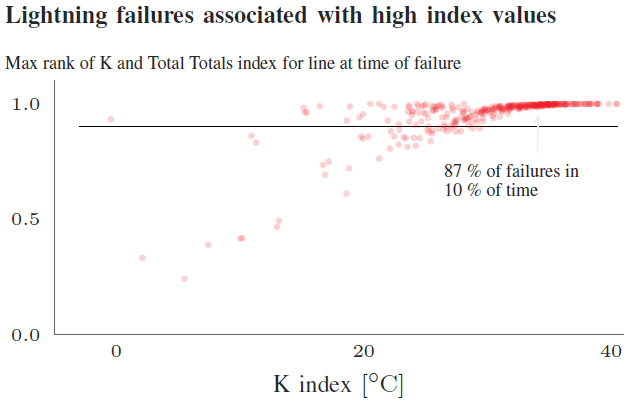Figure 1 Rank and K index for lightning failures

Figure 1 shows how lightning failures are associated with high and rare values of the K and Total Totals indices, computed from the reanalysis data set. For each time of failure, the highest value of the K and Total Totals index over the geographical span of the transmission line have been calculated, and then these numbers are ranked among all historical values of the indices for this line. This illustrates how different lines fail at different levels of the index values, but maybe even more important: The link between high index values and lightning failures is very strong. Considering all the lines, 87 percent of the failures classified as “lightning” occur within 10 percent of the time. This is promising…Figure 2: TT index versus K index shows seasonality trend

In Norway, lightning typically occurs during the summer in the afternoon as cumulonimbus clouds accumulate during the afternoon. But there is a significant number of failures due to thunderstorms during the rest of the year as well, winter months included. To see how the indices, K and T T , behave for different seasons, the values of these two indices are plotted at the time of each failure in Figure 3. From the figure it is obvious, though the data is sparse, that there is relevant information in the Total Totals index that has to be incorporated into the probability model of lightning dependent failures. The K index has a strong connection with lightning failures in the summer months, whereas the Totals Totals index seems to be more important during winter months.

Method in brief

The method is a two-step procedure: First, a long-term failure rate is calculated based on Bayesian inference, taking into account observed failures. This step ensures that lines having observed relatively more failures and thus being more error prone will get a relatively higher failure rate. Second, the long-term annual failure rates calculated in the previous step are distributed into hourly probabilities. This is done by modelling the probabilities as a functional dependency on relevant meteorological parameters and assuring that the probabilities are consistent with the failure rates from step 1.

Bayesian update

From the failure statistics we can calculate a prior failure rate$\lambda$ due to lightning simply by summing the number of failures per year and dividing by the total length of the overhead lines. We then arrive at a failure rate per 100 km per year. This is our prior estimate of the failure rate for all lines.

When we observe a particular line, the failures arrive in what is termed a Poisson process. When we assume that the failure rate is exponentially distributed, we arrive at a convenient expression for the posterior failure rate$\lambda^B$:$\lambda^B = \frac{1 + \sum{y_i}}{\frac{1}{\lambda} + n}$

Where$n$ is the number of years with observations,$\lambda$ is the prior failure rate and$y_i$ is the number of observed failures in the particular year.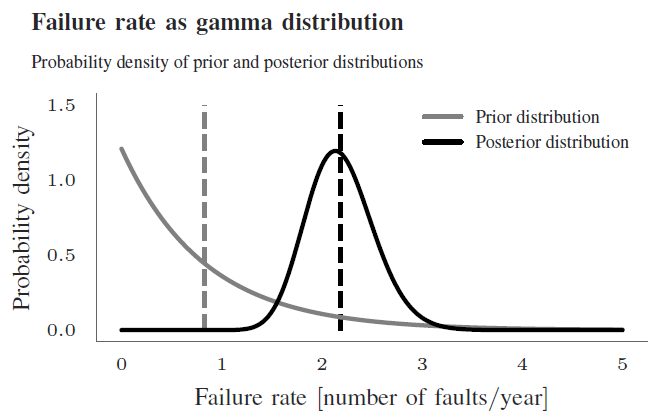Figure 3: The prior and the posterior distribution. Dashed vertical lines are theexpectations.

Distributing the long term failure rates over time

We now have the long-term failure rate for lightning, but have to establish a connection between the K-index, the Totals Totals index and the failure probability. The goal is to end up with hourly failure probabilities we can use in monte-carlo simulations of power system reliability.

The dataset is heavily imbalanced. There are very few failures (positives), and the method has to account for this so we don’t end up predicting a 0 % probability all the time. Read a good explanation of learning from imbalanced datasets in this kdnuggets blog.

Many approaches could be envisioned for this step, including several variants of machine learning. However, for now we have settled on an approach using fragility curves which is also robust for this type of skewed/biased dataset.

A transmission line can be considered as a series system of many line segments between towers. We assume that the segment with the worst weather exposure is representable for the transmission line as a whole.

We then define the lightning exposure at time$t$:$w^t = \alpha_K \max(0, K^t_{max} - K_{\text{thres}})^2 + \alpha_{TT} \max(0, TT^t_{max} - TT_{\text{thres}})^2$

Where$\alpha_K, \alpha_{TT}$ are scale parameters,$K_{max}^t$ is the maximum K index along the line at time$t$,$TT_{max}^t$ is the maximum Total Totals index at time$t$ along the line.$K_{\text{thres}}, TT_{\text{thres}}$ are threshold values for the lightning indices below which the indices has no impact on the probability.

Each line then has an probability of failure at time$t$ given by:$p_L^t = F(w^t; \sigma_L, \mu_L)$

where$F(\cdot)$ is the cumulative log normal function.

To find the standard deviation and expected value that describe the log normal function, we minimize the following equation to ensure that the expected number of failures equals the posterior failure rate:$\mu_L, \sigma_L = \underset{\mu, \sigma}{\text{argmin}} \: g(p^t_L; \mu, \sigma)$

where$g(p^t_L; \mu, \sigma) = \left(\lambda^B - \frac{1}{k}\sum_{t=0}^T p^t_L\right)^2$

If you want to delve deeper into the maths behind the method we will present a paper at PMAPS 2018.

Fitting the model to data

In this section simulation results are presented where the models have been applied to the Norwegian high voltage grid. In particular 99 transmission lines in Norway have been considered, divided into 13 lines at 132 kV, 2 lines at 220 kV, 60 lines at 300 kV and 24 lines at 420 kV. Except for the 132 and 220 kV lines, which are situated in Finnmark, the rest of the lines are distributed evenly across Norway.

The threshold parameters$K_{\text{thres}}$ and$TT_{\text{thres}}$ have been set empirically to$K_{\text{thres}} = 20.0$ and$TT_{\text{thres}} = 45.0$. The two scale parameters$\alpha_K$ and$\alpha_{TT}$ have been set by heuristics to$\alpha_K = 0.88$ and$\alpha_{TT} = 0.12$, to reflect the different weights of the seasonal components.

Results

The probability models presented above are being used by Statnett as part of a Monte Carlo tool to simulate failures in the Norwegian transmission system for long term planning studies. Together with a similar approach for wind dependent probabilities, we use this framework as the basic input to these Monte Carlo simulation models. In this respect, the most important part of the simulations is to have a coherent data set when it comes to weather, such that failures that occur due to bad weather appear logically and consistently in space and time.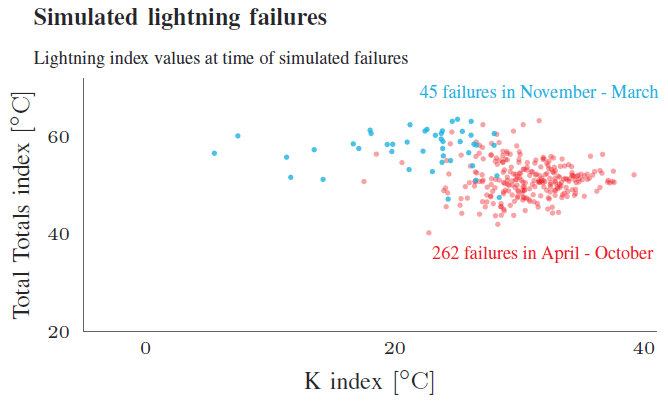Figure 4: Seasonal differences in K index and TT index for simulated results

Figure 4 shows how the probability model captures the different values of the K index and the Total Totals index as the time of the simulated failures varies over the year. This figure should be compared with figure 2. The data in Figure 4 is one out of 500 samples from a Monte Carlo simulation, done in the time period from 1998 to 2014.

The next figures show a zoomed in view of some of the actual failures, each figure showing how actual failures occur at time of elevated values of historical probabilities.